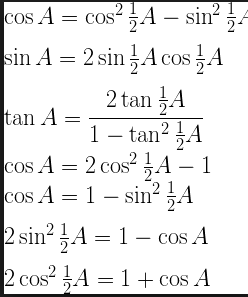# Ifis the arithmetic mean ofand, thenis  equal to : Option 1)Option 2)Option 3)Option 4)D Divya Saini

As we learnt in

Trigonometric Ratios of Submultiples of an Angle -- wherein

This shows the formulae for half angles and their doubles.

dividing  (2) by (1), we get

Thus,

Option 1)This option is incorrect.

Option 2)This option is incorrect.

Option 3)This option is correct.

Option 4)This option is incorrect.

Exams
Articles
Questions